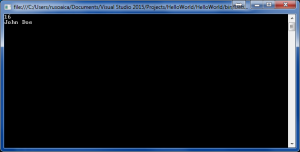Saturday, September 23, 2023 23:22

## Operators

No, we’re not talking about the landline phone operators 🙂 . As you can imagine, storing data in variables is pointless if we are not manipulating and/or using that data. Even in the most simple programs, you will use mathematical operations like subtraction, addition, multiplication, division. To perform these basic math functions, you will use operators.

Operators take as input one or more operands and return a value as a resultAs in math, operators have different precedence and higher/lower priority in regard one to another. Operators can be classified in a few categories:

• Arithmetic – they perform simple mathematical functions
• Comparison – used for comparing expressions or values
• Assignment – as their name suggest, they allow assignment of values to variables.
• Binary – they perform operations on the binary representation of the data
• Logical – they only work with Boolean values and expressions.
• Type conversion (casters) – they can be used to convert a data type to another data type.

Here is a list of operators, grouped by category:

You should know that the same operators applied on different data types produce different operations. For instance, the operator, when used on numerical data types (int, float, etc) performs mathematical addition. The same operator used with string variables will only concatenate (join together) the two strings.

The above source code will produce the following output, illustrating the different behavior of the + operator applied on different data types:The concepts explained in this lesson are also shown visually as part of the following video:

Tags: